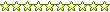View Single Post04-16-2004, 05:21 PM   #1
mike@af
Captain Over EngineerJoin Date: Feb 2003
Location: New York, New York
Posts: 6,711
Thanks: 27
Thanked 29 Times in 23 Posts
Fraction to Decimal Conversion Chart

This is a fraction to decimal conversion chart for all of you that still use the American English Standard Units.

Fractions in RED = Decimal in BLUE

1/64 = .0156
1/32 = .0312
3/64 = .0468
1/16 = .0625
5/64 = .0781
3/32 = .0937
7/64 = .1093
1/8 = .125
9/64 = .1406
5/32 = .1562
11/64 = .1718
3/16 = .1875
13/64 = .2031
7/32 = .2187
15/64 = .2343
1/4 = .250
17/64 = .2656
9/32 = .2812
19/64 = .2968
5/16 = .3125
21/64 = .3281
11/32 = .3437
23/64 = .3593
3/8 = .375
25/64 = .3906
13/32 = .4062
27/64 = .4218
7/16 = .4375
29/64 = .4531
15/32 = .4687
31/64 = .4843
1/2 = .500
33/64 = .5156
17/32 = .5312
35/64 = .5468
9/16 = .5625
37/64 = .5781
19/32 = .5937
39/64 = .6093
5/8 = .625
41/64 = .6406
21/32 = .6562
43/64 = .6718
11/16 = .6875
45/64 = .7031
23/32 = .7187
47/64 = .7343
3/4 = .750
49/64 = .7656
25/32 = .7812
51/64 = .7968
13/16 = .8125
53/64 = .8281
27/32 = .8437
55/64 = .8593
7/8 = .875
57/64 = .8906
29/32 = .9062
59/64 = .9218
15/16 = .9375
61/64 = .9531
31/32 = .9687
63/64 = .9843
1 = 1.000

Hope this helps all of you. I will add to this later Im tired of typing and stuff, I will add a conversion to metric (mm).
__________________
-Mike
AF Director of Media / Photographermike.lawrence@automotiveforums.com | AutomotiveForums.com | Flickr Gallery